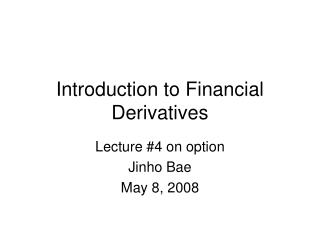DownloadDownload PresentationIntroduction to Financial Derivatives

# Introduction to Financial Derivatives

Download Presentation## Introduction to Financial Derivatives

- - - - - - - - - - - - - - - - - - - - - - - - - - - E N D - - - - - - - - - - - - - - - - - - - - - - - - - - -
##### Presentation Transcript

1. Introduction to Financial Derivatives Lecture #4 on option Jinho Bae May 8, 2008

2. Ch 8. Option pricing models I. Value of an option • Intrinsic value • Time value II. Factors that affect the price of an option

3. I. Value of an option • Value of an option =Option premium=Option price • The price that an option holder pays to an option writer for the right to sell or buy an asset • Value of an option= Intrinsic value + Time value

4. I-1-1. Intrinsic value of a call option • When the spot price (S) exceeds the strike price (X) Intrinsic value=S-X>0 e.g) Google call option with X=\$460 Google share price S=\$465 Intrinsic value=S-X=\$5

5. Intrinsic value of a call option • When the spot price (S) does not exceed the strike price (X) Intrinsic value=0 e.g) Google call option with X=\$460 Google share price S=\$450 Intrinsic value=0

6. Intrinsic value of a call option • Mathematical expression of intrinsic value of a call option max(S-X, 0) • When S>X, S-X>0  take S-X • When S<X, S-X<0  take 0

7. Intrinsic value of a call option value Intrinsic value X S

8. I-1-2. Intrinsic value of a put option • When the strike price (X) exceeds the spot price (S) Intrinsic value=X-S>0 e.g) Google put option with X=\$460 Google share price S=\$450 Intrinsic value=X-S=\$10

9. Intrinsic value of a put option • When the strike price (X) does not exceed the spot price (S) Intrinsic value=0 e.g) Google call option with X=\$460 Google share price S=\$465 Intrinsic value=0

10. Intrinsic value of a put option • Mathematical expression of intrinsic value of a put option max(X-S, 0) • When X>S, X-S>0  take X-S • When X<S, X-S<0  take 0

11. Intrinsic value of a put option value Intrinsic value X S

12. Relationship between intrinsic value and ITM, OTM, ATM

13. I-2. Time value of an option • The value of an option arising from the time left to maturity • Time value = Option premium - Intrinsic value e.g) IBM call option with X=\$100 trades at \$10 IBM share price S=\$106 Intrinsic value=S-X=\$6 Time value= \$10-\$6=\$4

14. Two elements of time value of an option • Time value 1: Expected payoff when holding the option until maturity 2) Time value 2: Time value associated with cash flow from selling or buying underlying asset of the option

15. Time value 1 Two scenarios of asset price movement until maturity • Asset price moves in a favorable direction  unlimited positive payoff • Asset price moves in an unfavorable direction  no or bounded loss Expected payoff is positive.

16. E.g) IBM call option, X= \$100, maturity=1 month ① current S=\$100 (ATM) • If ST (at maturity) > \$100  Payoff: ST - \$100 • If ST (at maturity) < \$100  No loss • Expected payoff from changes in the asset price until maturity > 0

17. Possibilities of changes in the asset price until maturity

18. ② current S=\$90 (OTM) • Intrinsic value=\$0 • If ST (at maturity) > \$100  Payoff: ST - \$100 • If ST (at maturity) < \$100  No loss

19. Expected payoff • Greater than 0. • However, smaller than that for ATM. Why?

20. ③ current S=\$110 (ITM) • Intrinsic value =\$10 • If asset price increases above 110  Payoff increases proportionally • If asset price increases below 110, intrinsic value decreases but bounded from 10.

21. Expected payoff • Greater than 0. • However, smaller than that for ATM.

22. Time value 1 of a call option X S value Time value 1 Current spot price OTM ATM

23. Time value 1 of a put option X S value Time value 1 Current spot price ATM OTM